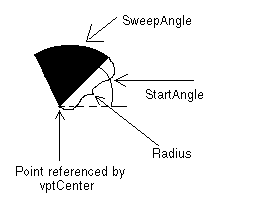﻿ VECTORARC | Vector Structures | Vector C API Help
In This Topic ▼

# VECTORARC

## Summary

The VECTORARC structure holds information about a vector arc object.

## Syntax

````typedef  struct  tagVECTORARC `
`{ `
`   VECTOROBJECT  Object; `
`   VECTORPOINT  Point; `
`   VECTORPEN  Pen; `
`   L_DOUBLE  Radius; `
`   L_DOUBLE  StartAngle; `
`   L_DOUBLE  SweepAngle; `
`}  VECTORARC,  *  pVECTORARC; ````

## Members

Abstract object.

### VECTORPOINT Point

A VECTORPOINT structure that contains the vertex of a vector arc object, about which the arc sweeps.

### VECTORPEN Pen

VECTORPEN structure that contains information about the pen to use when drawing the vector arc object.

Length of the radius of the vector arc object. This value is in logical units.

### L_DOUBLE StartAngle

The angle at which to begin the vector arc. Values should be between 0 and 360 degrees. Values less than 0 and greater than 360 will be modified internally.

### L_DOUBLE SweepAngle

The angle through which to sweep the vector arc. Values should be between 360 and +360 degrees. Positive angles will be drawn in a counter-clockwise direction from StartAngle. Negative angles will be drawn in a clockwise direction.

nSize of the Object member must be the sizeof this structure.

nType of the Object member must be VECTOR_ARC.

pVECTORARC is a pointer to a VECTORARC structure.

The diagram below gives a graphical representation of the Point, Radius, StartAngle and SweepAngle parameters.Help Version 22.0.2022.12.7